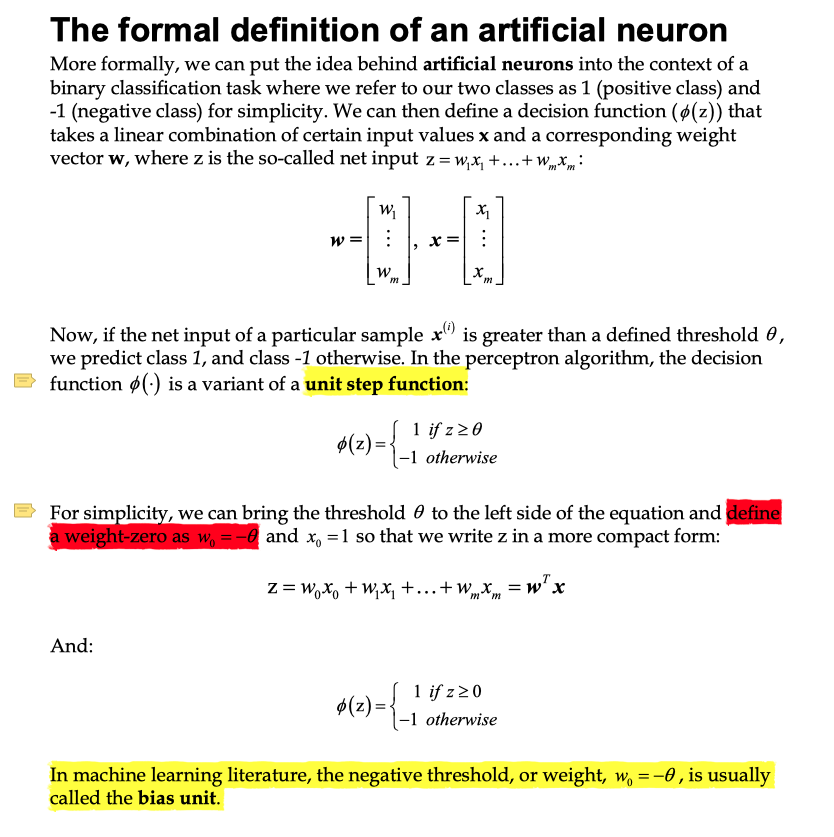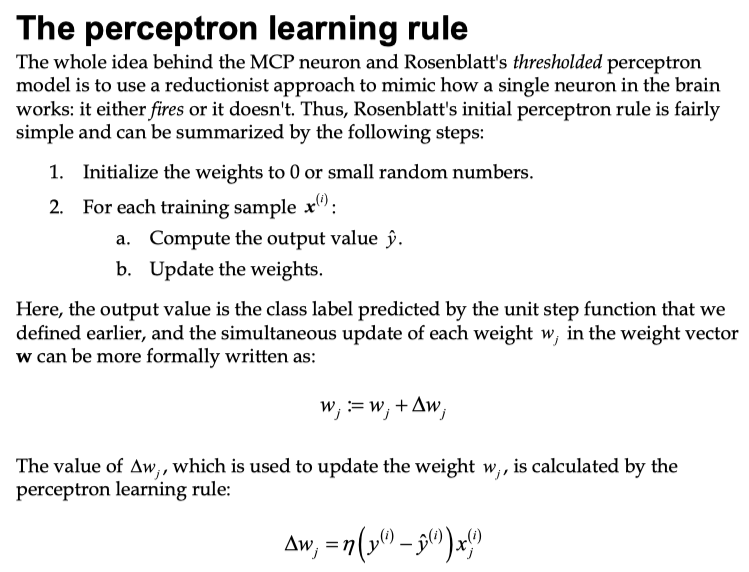神经网络概述

1. 样本集合可以近似线性分类的，否则我们会发现需要不停的来回调整权值
2. 学习速率尽可能小，否则同样会出现来回摇摆的情况。

神经网络感知机学习实现

Implementing a perceptron learning algorithm

算法实现

• Matplotlib: http://matplotlib.org/users/beginner.html

1. 初始化：ppn = Perceptron(eta=0.1, n_iter=10)
2. 调用 fit 方法：ppn.fit(X, y)

输入的数据

X：这是一个二维数组，其中使用了两个 feature，参考书第9页，也就是这里矩阵下标所表示的范围。一共有100个样本，也就是矩阵上标的范围, 矩阵可以表示为：

y：是一个一维数组，表示样本的实际分类。

初始权值

Via the fit method, we initialize the weights in self.w to a vector » m+1 , where m stands for the number of dimensions (features) in the dataset, where we add 1 for the first element in this vector that represents the bias unit. Remember that the first element in this vector, self.w, represents the so-called bias unit that we discussed earlier.

rgen.normal(loc=0.0, scale=0.01, size=1 + X.shape) 生成正态分布的随机数，loc 表示是分布的中心位置，scale 标准方差，用于描述分布的集中程度。最后一个参数表示输出的形态：X.shape 是2， 这里生成了3个数，其中下标为0的数是 bias unit

什么是 bias unit？使用样本修正权值

1. 初始化权值为 0 或者是一个很小的随机值
2. 然后根据训练的样本 $x^{(i)}$，和代价函数，计算预测的分类，然后根据实际的分类和学习速率更新权值数据处理 Pandas

从 CSV 中读取数据：